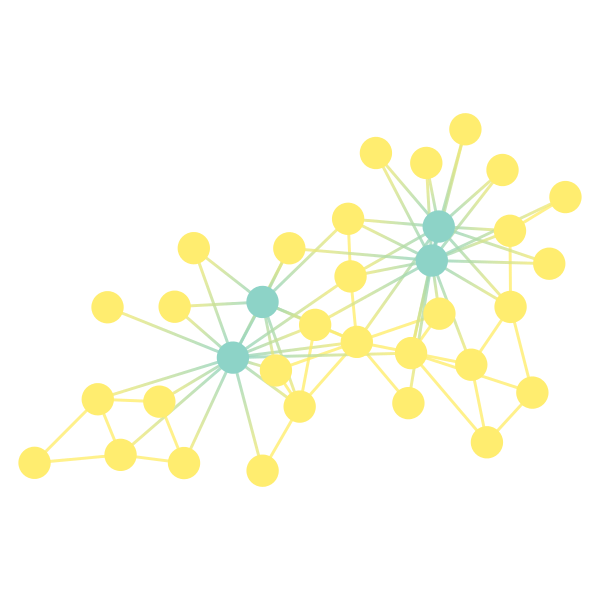# Inference for the "karate network"

Dear all,

Just as a test, I was trying to fit a degree-corrected SBM to the famous
"karate-club" network. Almost everybody has "shown" that the network
contains two communities. However, when I tried using in-built graph-tool
algorithms, I can see only one:

In : G = gt.collection.data["karate"]

In : state = gt.minimize_blockmodel_dl(G, deg_corr=True)

In : state
Out: <BlockState object with 1 blocks (1 nonempty), degree-corrected,
for graph <Graph object, undirected, with 34 vertices and 78 edges at
0xa288858c>, at 0xa288b9cc>

I tried this repeatedly, but I always get only one group. Then I tried
manually specifying 2 groups:

In : state = gt.minimize_blockmodel_dl(G, deg_corr=True, B_min=2)

However, in this case, I get a strange partition as shown in the following
figure:

This is not even close to what many papers show. I appreciate that many
algorithms consider noise as the real structure. However, even the famous
paper by Karrer and Newman (Karrer, Brian, and Mark EJ Newman. "Stochastic
blockmodels and community structure in networks." *Physical Review E* 83.1
(2011): 016107.) describes a very different division of the network. Am I
missing something very fundamental here?

Thank you
Snehal Shekatkar

attachment.html (2.17 KB)Dear Snehal,

I am not sure what others would say, but, in your case, I am pretty sure
that you have used the overlapping version of SBM. You will not observe the
core-periphery structure fitted by the vanilla SBM, nor the assortative
structure via the degree-corrected SBM.

Try:
state = gt.minimize_blockmodel_dl(G, deg_corr=True, overlap=False, B_min=2)
And visualize it via:
state.draw(pos=G.vp["pos"], vertex_shape=state.get_blocks())

You may get what you expected.

Also, note that if you tried (without the minimum block number constraint),
state = gt.minimize_blockmodel_dl(G, deg_corr=True, overlap=False)

You will still obtain a ground state with only one non-empty group. This is
valid since it is a state with the minimum description length (MDL) of both
the model and the data.
See this paper for details about MDL:
http://dx.doi.org/10.1103/PhysRevLett.110.148701

Note that people have argued whether it is necessary to introduce the
additional Order(N) parameters in the SBM to fit such a small karate club
data, which introduces a lot of model complexity. Indeed, if one used a full
Bayesian treatment and study the relation of the model likelihood to the
different numbers of blocks used. For the karate network, one may hesitate
which number of partitions to use.
See the Fig.2(a) of this paper:
https://journals.aps.org/prl/abstract/10.1103/PhysRevLett.117.078301

Finally, the karate network is not generated by any model of the SBM-family.
So it really takes interpretations between the model and the data!

Best regards,
Tzu-Chi Yen

Thanks for the reply. However, unfortunately this doesn't help to me. I
have seen those papers and I think the algorithm in the first paper is
in-built in graph-tool. Also, overlap = False is the default argument for
the minimize_blockmodel and hence what I was doing is no different than
what you said.

Snehal

attachment.html (3.54 KB)

Hmm. What about if the documentation indeed says overlap=True as the default?
https://graph-tool.skewed.de/static/doc/inference.html

Have you tried and observed a difference if you explicitly set "overlap" to
"False"? Does this help? If not, could you rephrase your question?

Bests,
Tzu-Chi Yen

Oops, this is a bug in the documentation. The default is indeed
overlap=False, as the function signature says. I'll fix it soon.

Best,
Tiago

Dear all,

Just as a test, I was trying to fit a degree-corrected SBM to the famous
"karate-club" network. Almost everybody has "shown" that the network
contains two communities. However, when I tried using in-built graph-tool
algorithms, I can see only one:

I don't think most of them have shown such a thing. Many methods _find_ two
communities, and many _assume_ that makes sense, but that is not _showing_
anything. Note that most methods would happily partition a fully random network.

I tried this repeatedly, but I always get only one group. Then I tried
manually specifying 2 groups:

In : state = gt.minimize_blockmodel_dl(G, deg_corr=True, B_min=2)

However, in this case, I get a strange partition as shown in the following
figure:

This partition is not strange, it is a division that separates the
administrator and instructor from the rest. Is one local maximum of the SBM
posterior. However, if you keep running the algorithm many times, you
eventually see the partition that you were expecting. (Remember that the
algorithm is stochastic).

If you look at the description length corresponding to each partition, you get:

B = 1, S = 222.6858
B = 2, S = 226.9818 (hubs vs. rest)
B = 2, S = 228.2842 (two communities, "conventional")

The posterior odds ratio of the B=1 vs the B=2 partitions is 0.014 and
0.004, so the B = 2 partitions cannot be fully rejected. This means that
there _is_ evidence for _any of the two_ partitions. However, it seems that
the most likely model is indeed a configuration model, B=1. And the only way
one could _show_ otherwise is to come up with a B>1 model that reduces the
description length below 222.6858 nats.

Note that the over-reliance on the karate club to judge the quality of
algorithms is highly questionable, and often the subject of jokes. See: